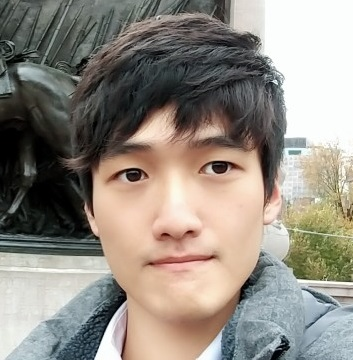Jaewoo Song

### Categories

• Tech

I found the data structure called “Priority Queue” in <queue> libaray.

This is literally a queue that contains data based on the priority, which is similar with a heap.

Let’s say that we use a priority queue for integer data.

#include <iostream>
#include <queue>
#include <vector>

using namespace std;

int main() {

priority_queue<int> q1;
priority_queue<int, vector<int>, less<int>> q2;
priority_queue<int, vector<int>, greater<int>> q3;

return 0;
}



We can say that q1 and q2 are the same structures.

When we declare priority_queue, we need the data type, structure and comparison operator as parameters.

If we specify only the type of data, which is int, then the structure becomes vector<int> and opertor is less<int>.

This gives more priority to bigger value by comparing in descending order.

If we want to make it in ascending order, we can put greater<int> to third parameter like in q3.

Now let’s see how the values are pushed and popped by exectuing below codes.

#include <iostream>
#include <queue>
#include <vector>

using namespace std;

int main() {

priority_queue<int> q1;
priority_queue<int, vector<int>, less<int>> q2;
priority_queue<int, vector<int>, greater<int>> q3;

vector<int> v = {3, 6, 1, 2, 0, 7};

for (int i=0; i<v.size(); ++i) {
q1.push(v[i]);
q2.push(v[i]);
q3.push(v[i]);
}

cout<<"q1: ";
while(!q1.empty()) {
cout<<q1.top()<<" ";
q1.pop();
}
cout<<"\n";

cout<<"q2: ";
while(!q2.empty()) {
cout<<q2.top()<<" ";
q2.pop();
}
cout<<"\n";

cout<<"q3: ";
while(!q3.empty()) {
cout<<q3.top()<<" ";
q3.pop();
}
cout<<"\n";

return 0;
}We can see that if the operator is declared as descending order, the values are ordered from bigger to smaller and vice versa.

Another interesting thing is that originally we use front() to check the front value of a queue, but instead we should use top() for a priority queue.

This is because a priority queue is more similar with a heap than with a original queue.

And the time complexity is also bounded by that of a heap.

It takes $O(logN)$ to push or pop a value, so it can be useful for problems which should be approached with $O(NlogN)$ time complexity.